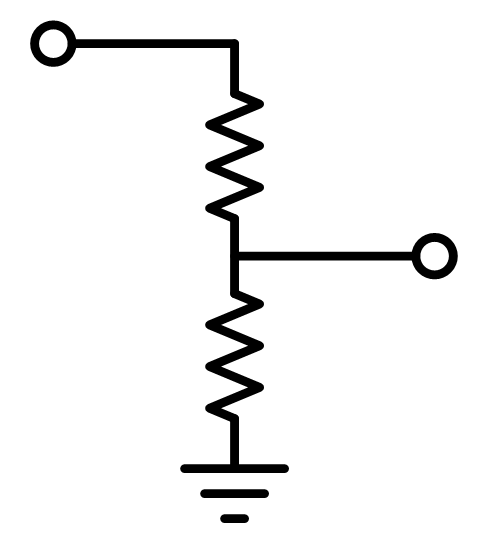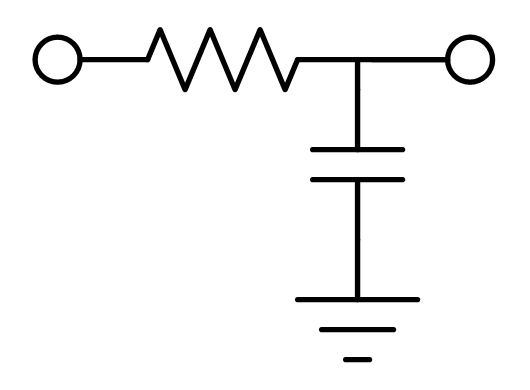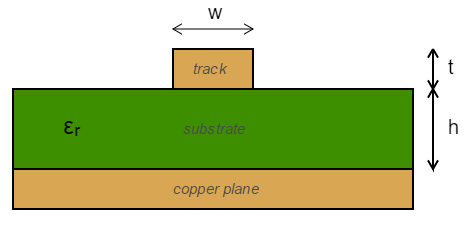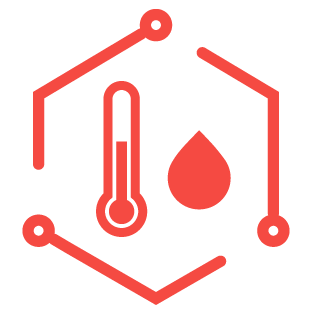• All (20)
• Electronics (17)
• Basics (5)
• Cabling (1)
• Filters (1)
• ICs (2)
• PCB Design (5)
• Sensors (2)
• SMPS (1)
• Mathematics (1)
• Geometry (1)
• Software (2)Calculate either the charge, capacitance or voltage across a capacitor using Q=CV.Calculate the value of a resistor needed to current-limit an LED.The hammer in any electrical engineers toolbox. Calculate voltage, resistance and current using Ohm's law.Resistor dividers are a simple, widely-used circuit primitive for reducing a voltage based on a fixed ratio.Find the closest E-series (e.g. E12, E96) resistor (preferred value) to your desired resistance.Find the right size wire gauge for a particular current and voltage drop.The low-pass RC filter is probably the simplist and most used electronic filter. Great for input signal filtering.Calculate the resistor and capacitors values for a 555 timer in astable configuration.A calculator to help you use the MP4558 IC in your next PCB. Calculates passive component values, frequencies, currents, e.t.c.A calculator for working out the impedance of a standard microstrip style track.PCB track current carrying capability calculator, using the IPC-2152 standard.PCB track current carrying capability calculator, using the IPC-2221a standard.PCB via current carrying capability calculator, using the IPC-2221A standard.Calculator the thermal resistance of a via.Calculate the dew point using the Magnus equation.Calculate the temperature, resistance (reference or actual), or beta-coefficient of NTC thermistors.This calculator can be used to calculate the values of the critical component values for a buck converter.Rotate objects in 3D using angle-axis, rotation matrices, euler angles or quaternions.Calculate CRC values from either a large number of popular CRC algorithms or define one yourself.